# MCQs on Electric Circuits

##### Page 22 of 63. Go to page 1 2 3 4 5 6 7 8 9 10 11 12 13 14 15 16 17 18 19 20 21 22 23 24 25 26 27 28 29 30 31 32 33 34 35 36 37 38 39 40 41 42 43 44 45 46 47 48 49 50 51 52 53 54 55 56 57 58 59 60 61 62 63
01․ When a lossy capacitor with a dielectric of permittivity ε and conductivity σ operates at a frequency ω the loss tangent for the capacitor is given by
ω σ/ε.
ω ε/σ.
σ/ω ε.
σ ω ε.

As C = Aε/d R = d/(σ A) CR = A ε/d d/(σ A) = ε/σ tanδ = I R/Ic = V/(R.Vω c) = I/(ω CR) So, tan δ = I(ω ε/σ) = σ/(ω ε).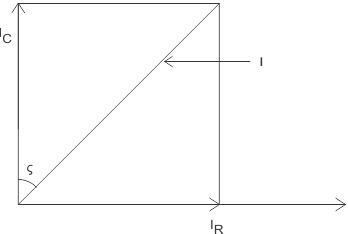02․ An ac voltage source v = vo sinωt is connected across a parallel plate capacitor C. If the conductor current is ic and the displacement current is id, then which is true among following relation
ic = id.
ic < id.
ic > id.
ic + id = 0.

The conduction current in the connecting wire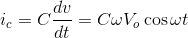For Parallel plate capacitor of area A and plate thickness d, C = ε A/d Electric field E in the dielectric is E = v/d. So, D = ε E = ε (Vo/d) sin ωt Displacement current id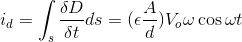Therefore, ic = id.

03․ The laplace transform of the fuction f(t) = t is
1/s.
s.
1/s2.
s2.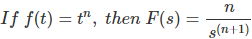04․ Conductance of any conductor is expressed as
ampere / watt.
mho.
volt2 / watt.
watt / ampere2.

Conductance is reciprocal of resistance that means conductance = (resistance) - 1 . Hence unit of conductance will be ( 1 / Ohm ) and this is known as mho.

05․ Three element having conductance G1, G2 and G3 are connected in parallel. Their combined conductance will be
(G1 + G2 + G3) - 1.
G1 + G2 + G3.
1 / G1 + 1 / G2 + 1 / G3.
(1 / G1 + 1 / G2 + 1 / G3) - 1.

We know that conductance is reciprocal of resistance i.e. resistance = 1 / conductance. Lets resistances of the said conductors are R1, R2 and R3.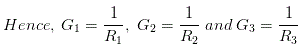The resistance of their parallel combination will be,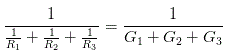Therefore, conductance of the combination will be G1 + G2 + G3.

06․ Which of the following materials has highest electrical conductivity ?
Steel.
Aluminium.
Copper.
Silver.

Silver has the highest conductivity among all other materials used as an electrical conductor. Electrical conductivity measures materials ability to conduct a current. It is commonly represented by the Greek letter σ’ (sigma). Its SI unit is siemens per metre.

07․ The electrical conductivity of metal is typically of the order of (in ohm - m)
107.
105.
10 - 4.
10 - 6.

The electrical conductivity of metal is typically of the order of 107 ohm - m.

08․ Pure metal generally have
high conductivity and low temperature coefficient.
high conductivity and large temperature coefficient.
low conductivity and zero temperature coefficient.
low conductivity and high temperature coefficient.

Pure metal always have high conductivity as well as high temperature coefficient. The resistance of metal greatly varies with temperature.

09․ Poorest conductor of electricity is
Aluminium.
Carbon.
Steel.
Silver.

Carbon is a non metal.

10․ Which variety of copper has the best conductivity ?
Pure annealed copper.
Induction hardened copper.
Hard drawn copper.
Copper containing traces of silicon.

Pure annealed copper has best conductivity.

<<<2021222324>>>